# Matching polynomial of a graph

(diff) ← Older revision | Latest revision (diff) | Newer revision → (diff)

A matching cover (or simply a matching) in a graphis taken to be a subgraph ofconsisting of disjoint (independent) edges of, together with the remaining nodes ofas (isolated) components. A matching is called a-matching if it contains exactlyedges. Ifcontainsnodes, then the extreme cases are:

i)is even and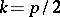; in this case, all the nodes ofare covered with edges (a perfect matching); and

ii)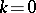; in this case, none of the nodes ofare covered by edges (the empty graph). If a matching containsedges, then it will have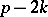component nodes. Now assign weights (or indeterminates over the complex numbers)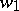andto each node and edge of, respectively. Take the weight of a matching to be the product of the weights of all its components. Then the weight of a-matching will be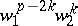. The matching polynomial of, denoted by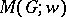, is the sum of the weights of all the matchings in. Setting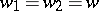, then the resulting polynomial is called the simple matching polynomial of.

The matching polynomial was introduced in [a1]. Basic algorithms for finding matching polynomials of arbitrary graphs, basic properties of the polynomial, and explicit formulas for the matching polynomials of many well-known families of graphs are given in [a1]. The coefficients of the polynomial have been investigated [a8]. The analytical properties of the polynomial have also been investigated [a10].

Various polynomials used in statistical physics and in chemical thermodynamics can be shown to be matching polynomials. The matching polynomial is related to many of the well-known classical polynomials encountered in combinatorics. These include the Chebyshev polynomials, the Hermite polynomials and the Laguerre polynomials. An account of these and other connections can be found in [a14], [a16]. The classical rook polynomial is also a special matching polynomial; and in fact, rook theory can be developed entirely through matching polynomials (see [a4], [a5]). The matching polynomial is also related to various other polynomials encountered in graph theory. These include the chromatic polynomial (see [a13]), the characteristic polynomial and the acyclic polynomial (see [a15] and [a3]). The matching polynomial itself is one of a general class of graph polynomials, called F-polynomials (see [a2]). Two graphs are called co-matching if and only if they have the same matching polynomial. A graph is called matching unique if and only if no other graph has the same matching polynomial. Co-matching graphs and matching unique graphs have been investigated (see [a6], [a9]). It has been shown that the matching polynomial of certain graphs (called D-graphs) can be written as determinants of matrices. It appears that for every graph there exists a co-matching D-graph. The construction of co-matching D-graphs is one the main subjects of current interest in the area (see [a7], [a11], [a12]).

How to Cite This Entry:
Matching polynomial of a graph. Encyclopedia of Mathematics. URL: http://encyclopediaofmath.org/index.php?title=Matching_polynomial_of_a_graph&oldid=11692
This article was adapted from an original article by E.J. Farrell (originator), which appeared in Encyclopedia of Mathematics - ISBN 1402006098. See original article